# Postfix Expression Evaluation

Evaluate the value of a Postfix expression, also known as expression in Reverse Polish Notation.
Given: An array of strings, every string being either an operand or an operator, in Reverse Polish Notation, find out the value of the expression.

Assumptions: The given postfix expression will be a valid expression.
1. String operands will be valid Integers (positive or negative)
2. Operators will be valid operators in: + - / *
3. 0 will not be passed as a divisor for division operation.

Example:
Input:
20    30    +
Output:
50

Input:
5    1    2    +    4    *    +    3    -
Output:
14

Note: Input is an array of strings to allow numbers with multiple digits as operands.
For example "20", "45" etc.

## Algorithm/Insights

Reverse Polish notation (RPN) is a mathematical notation in which every operator is placed after its operands. For example, x + y (in infix notation) is expressed in RPN as x y +
It is also known as postfix notation.

The expression can be evaluated using a stack as described below:
1. If the array has only 1 element, then return the element.
2. Traverse the string array for elements i = 0 to length of array:
a. If the element at index i is an operand, then parse it to get the value.
b. Else, pop two elements from the stack (say x and y) and perform the operation specified by the operator (for example, +) on the 2 elements in the below order and store it as the value:
y = s.pop()
x = s.pop()
value = x + y
c. We will get a value from steps a OR b, push the value on to the stack.
3. Finally, only 1 integer element is left on the stack, return it.

Example:
String Array:    5    1    2    +    4    *    +    3    -

1. Initialize empty stack.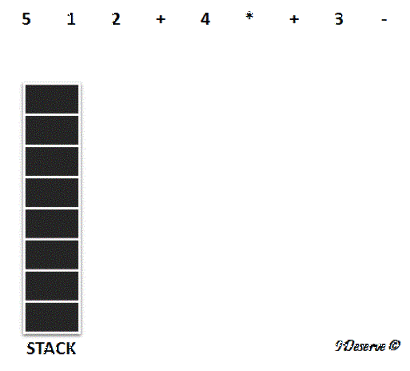2. Since 5 is an operand, add it to the stack.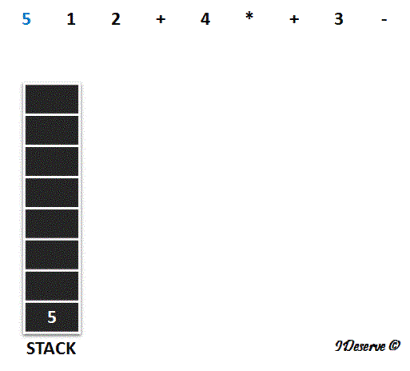3. 1 and 2 are also operands, so add these to the stack too.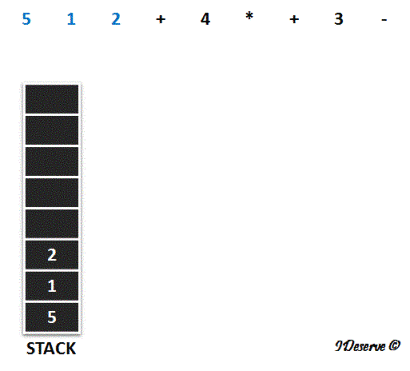4. Next we have + operator,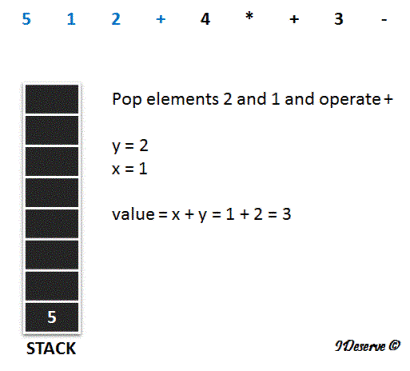5. Push the value onto the stack.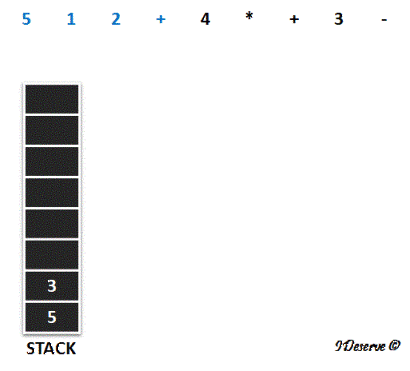6. Next we have 4, which is an operand, so push it to the stack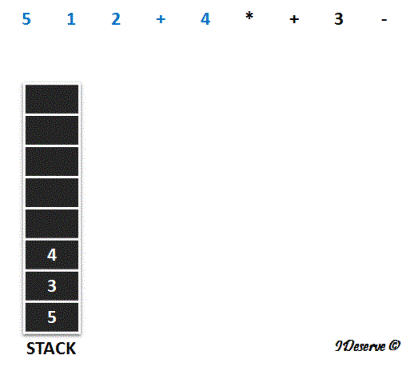7. Next we have * operator,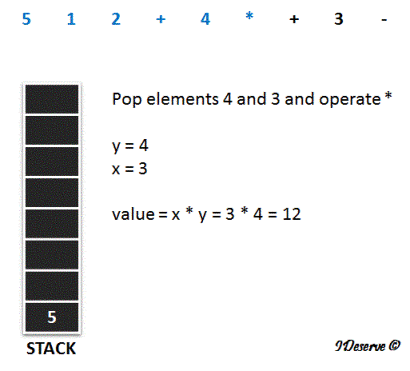8. Push the value onto the stack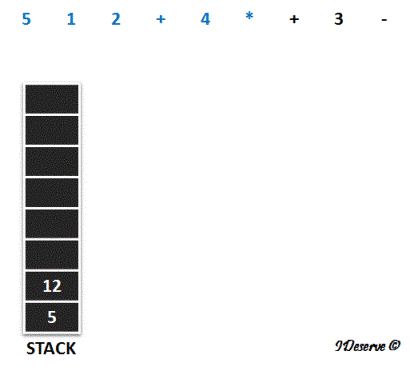9. Next we have + operator,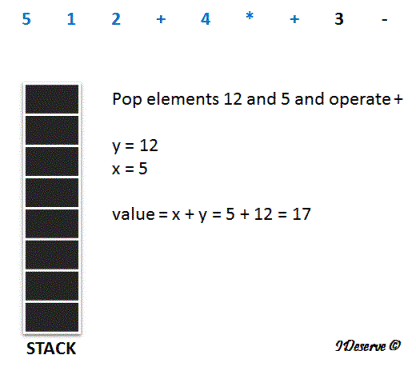10. Push the value 17 onto the stack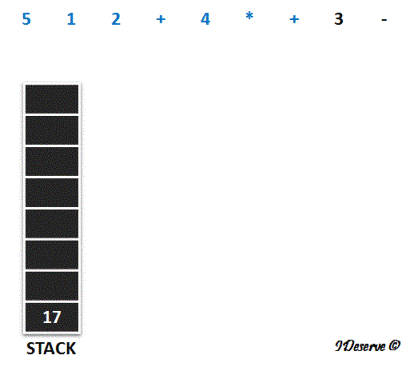11. Next element is an operand 3, push it to the stack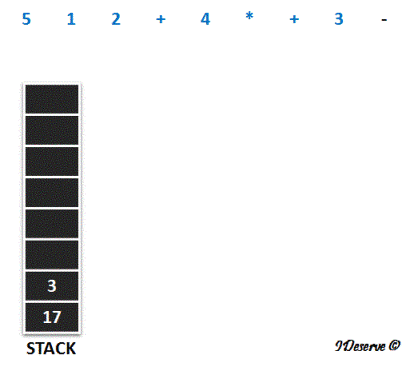12. Last, we have - operator,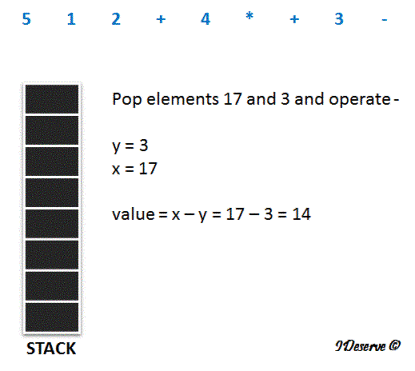Finally, all the array elements are processed, and we have 14 as the result.

## Code Snippet

```
package com.ideserve.questions.saurabh;

import java.util.Stack;

/**
* <b>IDeserve <br>
* Given an array of Strings, every string being either an operand or an operator,
* in Reverse Polish Notation, find out the value of the expression.
*
* @author Saurabh
*/
public class PostfixExpressionEvaluation {
public static int evalPostfixExpression(String[] op) {

if(op.length == 1) {
return Integer.parseInt(op);
}

Stack<Integer> s = new Stack<Integer>();
for(String exp : op) {

Integer value = null;
String operator = null;
if((exp.charAt(0) >= '0' && exp.charAt(0) <= '9') ||
(exp.charAt(0) == '-' && exp.length() > 1)) {
value = Integer.parseInt(exp);
} else {
operator = exp;
}

if(operator != null) {
Integer y = s.pop();
Integer x = s.pop();

switch(operator) {
case "+" : value = x + y; break;
case "-" : value = x - y; break;
case "*" : value = x * y; break;
case "/" : value = x / y; break;
}
}
s.push(value);
}
return s.pop();
}

public static void main(String[] args) {
String[] op = {"5", "1", "2", "+", "4", "*", "+", "3", "-"};
System.out.println("Value of the expression is " + evalPostfixExpression(op));
}
}
```

## Order of the Algorithm

Time Complexity is O(n)
Space Complexity is O(n)